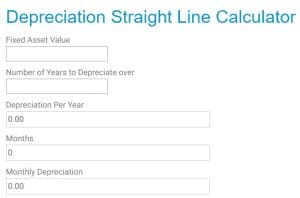# Free Depreciation Calculator Online

##How is Depreciation Calculated?

Use our free online depreciation calculator to work out the depreciation of the fixed assets for your business. Depreciation is a way to reduce an asset value over a longer period of time. An example is over three or five years.

Assets of a business have a useful life, and you will need to know either how many years to depreciate or the percentage.

There are two main methods which are used. If you are unsure which way to use, have a look at straight-line depreciation and reducing balance depreciation.

The straight-line method reduces the balance by the same amount each year and is the most common method. The reducing method reduces the amount by a percentage each year. The following year it reduces from the end balance from the previous year.

When you have a depreciation method in place, you should continue to use the same process for all the assets, unless an accountant has advised otherwise.

## Straight Line Method of Depreciation Calculator

If you are using the straight-line depreciation method and want to calculate for more than one asset, why not download our free Excel template depreciation schedule.## Depreciation Calculators Instructions

To use this calculator first enter the cost price of the fixed asset, then enter the number of years you are planning to use the asset over.
If your calculation requires a residual value at the end of the asset life, you will need to reduce the asset value by the residual value and enter that figure.

The calculator results will show you the depreciation per year and month. Post these figures as a journal to your accounts; this will reduce your asset value and increase the expenses.

## Reducing Balance Depreciation Calculator

If you are unsure of the depreciation percentage to use for depreciation, you will need to speak to an accountant to confirm the figure.

To use the calculator, you will need to enter the value of the asset and then the percentage of depreciation for each year.

If your asset needs depreciating for more than 5 years, calculate the first 5 years. Take the final written down value from year 5 and enter it to the asset value. It will then calculate the next 5 years.

Below shows the difference between the straight line and reducing balance depreciation. As you can see the straight line is consistent each year, while the reducing balance depreciates more in the early years.Return from depreciation calculator to depreciation page.